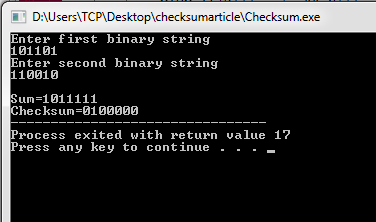# Checksum Program in C and C++

Here you will get checksum program in C and C++.

A checksum is a error detection method in Data Communication. It is used for errors which may have been introduced during transmission or storage. It is usually applied to an installation file after it is received from the download server.

The actual procedure which yields the checksum, given a data input is called a checksum function or checksum algorithm.

Also Read: Hamming Code in C and C++

Checksum method can only detect errors but is unable to correct the error.

In this method a checksum is calculated based on the given binary strings which is sent with the data as redundant bits. This data + checksum is received at receiver end and checksum is calculated again, if checksum is 0 it means no error in data received, else there exists some error in the received data.

For the purpose of this program we are finding checksum for 2 binary strings.

### Checksum Algorithm

1. Take 2 binary input strings.
2. Do their binary sum to find out the checksum which will be sent to the destination or to the receiver.
3. In binary sum there are 6 cases:-
1. If both bits are 0 and carry is 0, sum=0 and carry=0
2. If both bits are 0 and carry is 1,sum=1 and carry=0
3. If both bits are 1 and carry is 0,sum=0 and carry=1
4. If both bits are 1 and carry is 1,sum=1 and carry=1
5. If either bit is 1 and carry is 0,sum=1 and carry=0
6. If either bit is 1 and carry is 1,sum=0 and carry=1
4. While doing the addition we have to add the binary strings from rightmost end i.e LSB to MSB.
5. When binary sum is done 1’s complement of it is taken by reversing 1’s to 0’s and vice versa.
6. The resulting 1’s complement is the Checksum.
7. Stop.

## Checksum Program in C++

OutputComment below if you have queries or found anything incorrect in above checksum program in C and C++.

### 6 thoughts on “Checksum Program in C and C++”

1.Generally, if msb of sum is 1 then we need to add 1 to resultant sum but in above algorithm you didn’t implement it.
Can you please tell me that not doing so would make no difference…

2.No it’s wrong… You have to take the data as input and also the k value and you also didn’t add the carry to the sum which should be done according to the algorithm.

3.very very thanks

4.anyone send correct code

1.did you get the correct answer?

5.Sainik Kumar Mahata

Code is wrong, the anser has 7 bits, while the inputs have 6 bits. You have to add the extra MSb to the whole result.